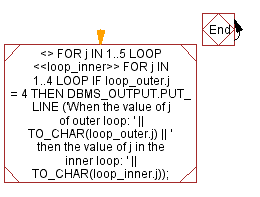﻿ PL/SQL Control Statement: Explain the uses of nested for loop with label - w3resource# PL/SQL Control Statement Exercises: Explain the uses of nested for loop with label

## PL/SQL Control Statement: Exercise-26 with Solution

Write a program in PL/SQL to explain the uses of nested for loop with label.

Sample Solution:

PL/SQL Code:

``````BEGIN
<>
FOR j IN 1..5 LOOP
<<loop_inner>>
FOR j IN 1..4 LOOP
IF loop_outer.j = 4 THEN
DBMS_OUTPUT.PUT_LINE
('When the value of j of outer loop: ' || TO_CHAR(loop_outer.j) || ' then the value of j in the inner loop: '
|| TO_CHAR(loop_inner.j));
END IF;
END LOOP loop_inner;
END LOOP loop_outer;
END;
/
```
```

Flowchart:Sample Output:

```When the value of j of outer loop: 4 then the value of j in the inner loop: 1
When the value of j of outer loop: 4 then the value of j in the inner loop: 2
When the value of j of outer loop: 4 then the value of j in the inner loop: 3
When the value of j of outer loop: 4 then the value of j in the inner loop: 4

PL/SQL procedure successfully completed.
```

Improve this sample solution and post your code through Disqus

What is the difficulty level of this exercise?

﻿